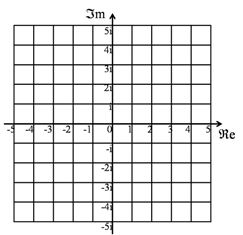The Argand Diagram is a geometric representation of Complex Numbers. The real number part is taken as a distance along the X-axis, the imaginary number part is taken as the distance along the Y-axis, giving a point in two dimensional space.

Addition of complex numbers can be thought of as vector addition, and multiplication is done by multiplying the lengths of the vectors, and adding the angles (taken positive in an anti-clockwise direction from the X-axis).